# Optimal programming control

(diff) ← Older revision | Latest revision (diff) | Newer revision → (diff)

A solution of a problem in the mathematical theory of optimal control (cf. Optimal control, mathematical theory of), in which the controlis formed as a function of time (whereby it is also supposed that, during the process, no information, apart from that which is given at the very beginning, is obtained). In this way, an optimal programming control is formed through a priori information on the system and cannot be corrected, unlike an optimal synthesis control.

The problem of existence of solutions to a problem of optimal programming control breaks down into two questions: explaining the possibility of realizing the aim of the control with the given constraints (the existence of an admissible control which realizes the aim of the control) and establishing the solvability of an extremum problem — attainability of the (as a rule, relative) extremum — in the aforementioned class of admissible controls (the existence of an optimal control).

It is particularly important, in relation to the first question, to study the property of controllability of a system. For a system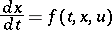it signifies the existence in a given classof admissible control functionswhich transfer a phase point (see Pontryagin maximum principle) from any given starting position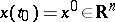to any given final position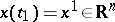(for a fixed or free time, dependent on the formulation of the problem). The necessary and sufficient conditions for controllability (or for complete controllability) are known in computable form for linear systems(1)

with analytic or periodic coefficients (these are simplest when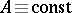,). For general linear systems the question of solvability of the problem of transfer from one convex set onto another (with convex constraints on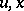) has also been completely solved. In non-linear systems, only local conditions of controllability (in a small neighbourhood of the given trajectory) or conditions for particular classes of systems (see , , ) are known. The property of controllability has also been studied for numerous generalizations relating, in particular, to special classes(for example, the setof all bounded, piecewise-continuous controls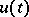), for controllability of some of the coordinates, or for more general classes of systems, including infinite-dimensional ones.

The question of the existence of an optimal control is in general related to a compactness property, in some topology, of minimizing sequences of controls or trajectories, and to the property of semi-continuity in the corresponding variables of the minimizing functionals. For a system(2)

under the constraints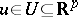(3)

the first of these properties is associated with convexity of the set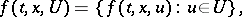while the second (for integral functionals) is linked with convexity in the corresponding values of. The lack of these properties is compensated for by broadening the initial variational problems. So, the non-convexity ofcan be compensated for by the introduction of sliding systems: Generalized solutions of ordinary differential equations, generated by control-measures given onand creating an effect of "convexification" (see , ; cf. also Optimal sliding regime). The absence of convexity in integral functionalsis compensated for by imbedding the problem in a more general one, with a new functional which is a convex minorant of the previous one, and imbedding the solution of the new problem in a broader class of controls (see ). In the instances shown, the existence of an optimal control often follows from the existence of an admissible control.

The theory of necessary conditions for an extremum is most developed in problems of optimal programming control. The Pontryagin maximum principle has served as a basic result in this case, as it includes necessary conditions for a strong extremum in a problem of optimal control.

General methods for obtaining necessary conditions in extremum problems were created and are used effectively for problems of optimal programming control with more complex constraints (such as phase, functional, minimax, mixed, etc.). They are based, in one way or another, on theorems of separability of convex cones (see , ). For example, letbe a vector space, let,, be a given functional, letbe a set from, let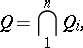let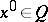be a point at whichreaches its minimum on, and letThe essence of one wide-spread general method is that every one of the sets,, is approximated in a neighbourhood of the point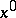by a convex conewith vertex at(the "descent cone" for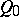; the cone of "admissible directions" for constraints of inequality type; the cone of "tangent directions" for equality-type constraints, including differential links, etc.). A necessary condition for a minimum is now thatis the single point common to all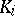,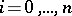, and, consequently, that the cones are "separable" (see ). An analytic form is added to the latter "geometric" condition and, where possible, is put into an appropriate form, for example, using a Hamilton function. Dependent on the initial constraints, as well as on the class of applicable variations, necessary conditions can take both a form analogous to the Pontryagin maximum principle and a form of a local (linearized) maximum principle (of a condition of a weak extremum with respect to). The realization of this method thus depends on the possibility of describing the conesanalytically. Their effective description has been achieved for sets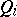defined by smooth functions which satisfy certain supplementary regularity conditions at the examined point or by convex functions (see , ). In principle, this method also permits generalizations to the case of non-smooth constraints, including differential ones. Here, for example, the concept of a subdifferential of a convex function and its generalization can be used, when convexity is lacking (see , ).

Conditions of the first order, analogous to the Pontryagin maximum principle, are known for solutions in the class of generalized function-measures (the so-called integral maximum principle), for controllable systems which can be described by differential equations with a perturbed argument, partial differential equations, evolution equations in a Banach space, differential equations on manifolds, recurrence difference equations, etc. (see , , , ).

From the necessary conditions for an extremum given in a problem of optimal control follow the well-known necessary first-order conditions of the classical variational calculus. In particular, in a two-point boundary value problem for the systems (2) and (3), whereis an open set,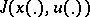is a standard integral functional, the Pontryagin principle implies the Weierstrass necessary condition for an extremum in the classical variational calculus.

Methods are being developed in the theory of optimal control to obtain necessary higher-order conditions (especially, of the second order) for non-classical variational problems (see ). Interest in higher-order conditions has largely been related to the study of degenerate problems of optimal control, leading to the so-called special controls, which do not have adequate analogues in the classical theory. For example, in the Pontryagin principle, the function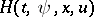can either lead to a whole family of controls, each of which satisfies the maximum principle, or does not depend onat all (in which case any of the admissible values ofsatisfies the Pontryagin principle). This situation is quite characteristic of a whole series of applied control problems in space. In the given case, the isolation of an optimal control already requires a mastering of the first-order extremals (the so-called Pontryagin extremals) and the use for them of necessary second-order optimality conditions (or, in general, those of higher order). Different forms of necessary conditions have been obtained here by the use of special classes of "non-classical" variations (for example, a "bundle" of needle variations, etc.). The realization of special controls is also often connected with the use of sliding systems (see , ).

The theory of sufficient conditions of optimality has not been examined in much detail. Results are known which relate to conditions of local optimality and contain, among other simple requirements, conditions of non-degeneracy of the variational system and constraints on the properties of the Hessian of the right-hand sides, calculated along an admissible trajectory for the corresponding ordinary differential equation. Another group of sufficient conditions is based on the method of dynamic programming and its relation to the theory of the maximum principle (see ). There are also formalisms which lead to sufficient conditions for an absolute minimum, based on the idea of broadening variational problems. Their domain of practical applicability embraces special classes of problems with convexity criteria and degenerate problems of optimal control (see ).

The complete solution of a problem of optimal programming control (necessary and sufficient conditions of optimality) is known for linear systems (1) when both the functionals and constraints onare convex (in a number of cases certain extra conditions have to be fulfilled here). The concept of duality, used in convex analysis, has demonstrated a particular extremal property of the trajectories of the system, described by conjugate variables in the Pontryagin maximum principle. This has enabled one to reduce the boundary value problem arising from the application of necessary conditions of general type to a solution of a simpler dual extremal problem. Within the framework of this approach the theory of linear systems with impulse controls has been developed, which simulates objects subject to instantaneous influences (percussion, explosive, impulse), and which is formalized by the use of differential equations in generalized functions with corresponding orders of singularity. The method of attainability domains (see , ) has been put to effective use, especially in the theory of game systems.

In the absence of complete a priori information on the system (including the statistical description of insufficient quantities) the problem of optimal programming control is studied under conditions of uncertainty. In the system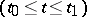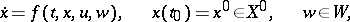(4)

let the parameter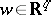, realized in the form of time functions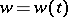, and the vectorbe unknown, and let only the sets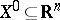,be given. Then, assuming the existence and extendability to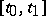of the solutions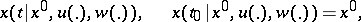of equation (4) (for given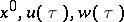,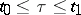), a bundle (ensemble) of trajectories can be formed:By selecting a programming control(the same one for all trajectories of the bundle), it is possible to control the position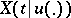in the phase space. A typical problem of optimal programming control under conditions of uncertainty consists of the optimization ofalong a functionalof maximum type:(5)

(then the solutionof the problem will ensure a guaranteed result) or of an integral functional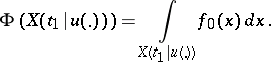(6)

The use of the technique of inferring necessary conditions of optimality or its modifications has enabled one to formulate requirements which ensure the existence of analogues of the Pontryagin principle for the problems (5), (6) (in the first instance it takes the form of a minimax condition). For linear systems, these problems allow just as detailed a solution as for systems with complete information (see , , ).

How to Cite This Entry:
Optimal programming control. Encyclopedia of Mathematics. URL: http://encyclopediaofmath.org/index.php?title=Optimal_programming_control&oldid=13851
This article was adapted from an original article by A.B. Kurzhanskii (originator), which appeared in Encyclopedia of Mathematics - ISBN 1402006098. See original article